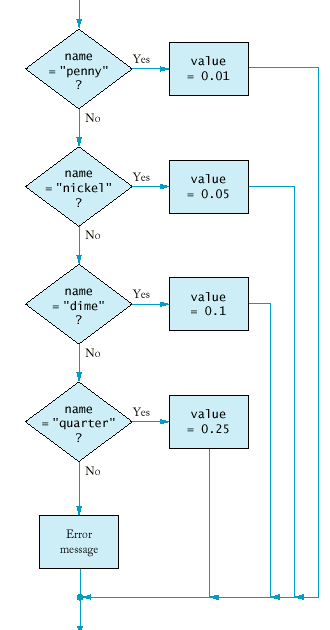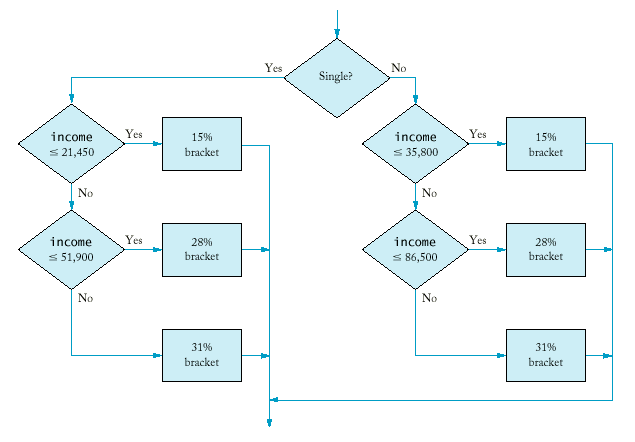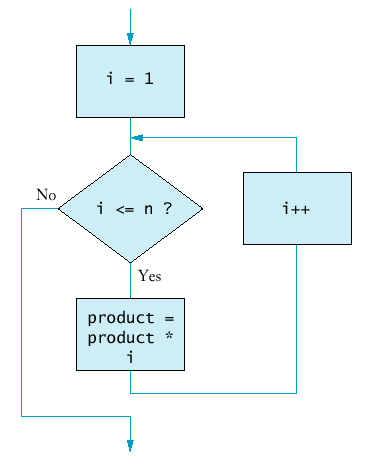## Chapter Goals

• To recognize the correct ordering of decisions in multiple branches
• To program conditions using Boolean operators and variables
• To understand nested branches and loops
• To be able to program loops with the for statement

## Multiple Alternatives

• By using collections of if/else statements, a program can distinguish between multiple alternatives.
• Example: The user enters the name of a coin, and the program returns the value.
• This program has five alternatives to choose from:
• "penny"
• "nickel"
• "dime"
• "quarter"
• erroneous input
• The order that the alternatives are checked is unimportant.

## Multiple Alternatives (Coins Flowchart)## Multiple Alternatives (coins5.cpp)

• In some cases, the order of the tests is important.
• Example: A program that displays a description of the likely impact of an earthquake based on its magnitude on the Richter scale.

## Multiple Alternatives (richter.cpp)

• Note that the order of the tests ensures that the right results are printed (reverse the order of tests).
• Note that the if/else structure ensure the alternatives are exclusive. Independent if statements may cause a single input to print several messages ().

## Nested Branches

• Nested if/else statements can be used when there are two (or more) levels of decision making.
• Example: Taxes
• There is a different table for each marital status (decision 1).
• Once you have found the right table, your are taxed differently according to your income (decision 2).

 If your status is Single and if the taxable income is over but not over the tax is of the amount over \$0 \$21,450 15% \$0 \$21,450 \$51,900 \$3,217.50 + 28% \$21,450 \$51,900 \$11,743 + 31% \$51,900

 If your status is Married and if the taxable income is over but not over the tax is of the amount over \$0 \$35,800 15% \$0 \$35,800 \$86,500 \$5,370.00 + 28% \$35,800 \$86,500 \$19,566.00+ 31% \$86,500
• The two-level decision process is reflected in two levels of if statements.
• We say the income test is nested inside the test for filing status.• More complicated decisions may require deeper levels of nesting.

## Boolean Operations

• An operator that combines test conditions is called a logical operator.
• The && (and) operator combines several tests into a new test that passes only when all the conditions are true.
• Example: Suppose you want to test whether your homework is due right now. The test passes only if both fields match.
`if (now.get_hours() == homework.get_hours() &&    now.get_minutes() == homework.get_minutes())       cout << "The homework is due right now!\n";`
• hwdue1.cpp
• The || (or) operator combines two or more conditions and succeeds if at least one of the conditions is true.
• Example: A test whether an order is shipped to Alaska or Hawaii.
`if (state == "HI" || state == "AK")    shipping_charge = 10.00;`
• hwdue2.cpp• The && and || operators are computed using lazy evaluation: the expressions are evaluated from left to right, and evaluation stops as soon as the truth value (true or false) is determined.
`if (cin.fail() || area < 0) cout << "Input error.\n";	if (r >= 0 && -b / 2 + sqrt(r) >= 0) ...`
• The ! (not) operator takes a single condition and evaluates to true if that condition is false and to false if that condition is true.
`if (!cin.fail()) quarters = quarters + n;`
• Summary:

 A B A && B true true true true false false false Any false

 A B A ||B true Any true true false true false false false

 A !A true false false true

## DeMorgan's Law

• Humans generally have a hard time comprehending logical conditions with not operators applied to and/or expressions.
• Example: Suppose we want to charge a higher shipping rate if we don't ship within the continental United States.
`if (!(country == "USA" && state != "AK" && state != "HI"))                      shipping_charge = 20.00;`
• Is it not true that some people won't be confused by this code?
• DeMorgan's Law can be used to simplify these Boolean expressions.
• DeMorgan's Law has two forms:

 not (A and B) is the same as not A or not B not (A or B) is the same as not A and not B
• Pay particular attention to the fact that the and and or operators are reversed by moving the not inwards.
• Example:
`state != "AK" && state != "HI"`
means
`!(state == "AK") && !(state == "HI")`
Applying DeMorgan's Law to the original expression yields
`!(state == "AK" || state == "HI")`
Finally we have (lazy evaluation!)
`if (country != "USA" || state == AK" || state == "HI") ...`

## The for Loop

• The most common loop has the form
`i = start;while (i <= end){  . . .    i++;}`
• Because this loop is so common, there is a special form for it.
`for (i = start; i <= end; i++)`
• Example: Let us compute the function factorial, n! = 1.2.3.4...n.
`int product = 1;for (int i = 1; i <= n; i++) product = product * i;`## Syntax 7.1: for Statement

Syntax 7.1 : for Statement

`for (initialization_expression; condition; update_expression) statement`
 Example: `for (int i = 1; i <= 10; i++) sum = sum + i;` Purpose: Execute the initialization statement. While the condition remains true, execute the statement and update the expression.

## The for Loop (forfac.cpp)

• We can count down instead of up:
`for (int n = 10; n >= 0; n--) ...`
• The increment or decrement need not be in step 1:
`for (x = -10; x <= 10; x = x + 0.5) ...`
• It is possible to put unrelated conditions into the loop (bad taste):
`for (rate = 6; month--; cout << balance) ...`